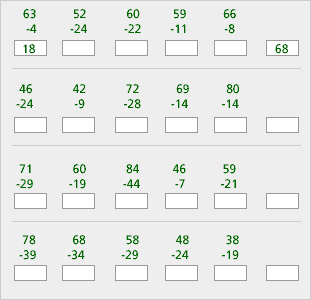# Classroom Case Studies, Grades K-2 Part D: Critiquing Student Lessons (20 minutes)

## Session 10: K-2, Part D

In the K-2 curriculum, students are frequently asked to think about patterns, but often their “pattern sniffing” skills end with simply finding the next object. Note 7

Here is a “pattern” problem.

Subtract, then look at the differences in each row.
Continue the pattern.### Problems

Problem D1

What algebraic ideas are in this lesson?

Problem D2

How are patterns used in this lesson?

Problem D3

What mathematics do you think students would learn from this lesson?

Problem D4

Are there any misconceptions that students might develop from this lesson?

Problem D5

How would you modify the problem, or what additional questions might you ask, to incorporate the framework for analyzing patterns?

### Notes

Note 7

You may be puzzled by this series of subtraction problems, as well you should! After working on Problems D1-D5, you may notice that in certain problems — where students “see” the pattern and then mindlessly fill in numbers that satisfy the pattern — the mathematical thinking and reasoning gets lost.

### Solutions

Problem D1

Problem D2

Answers will vary. The patterns shown only relate to the answers. For the first row, the answers increase by 10, but the problems themselves are not directly related. The second row’s answers are consecutive multiples of 11, but the problems are not related. The third row’s answers decrease by one with unrelated problems, and the fourth row’s answers decrease by five with unrelated problems.

Problem D3

Answers will vary. Students should strengthen their subtraction with regrouping skills.

Problem D4

Answers will vary. Students could learn to look for patterns in answers without actually looking at the problems. The answers may not have any pattern.

Problem D5

Answers will vary. One way to modify the problem would be to make the problems relate to the pattern of the answers. For example, the first row could show 63 – 55, 63 – 45, 63 – 35, 63 – 25, 63 – 15, then ask what comes next.